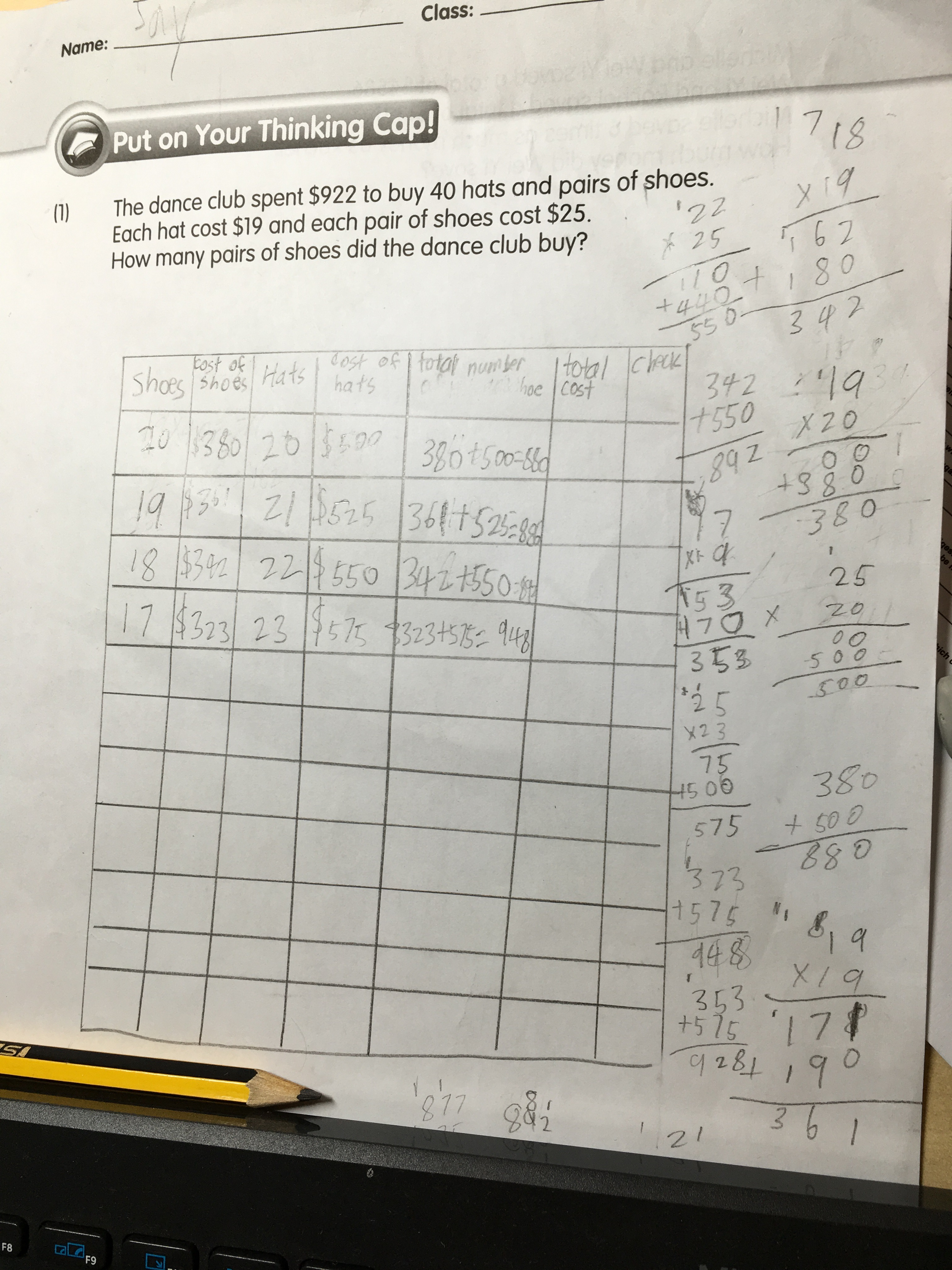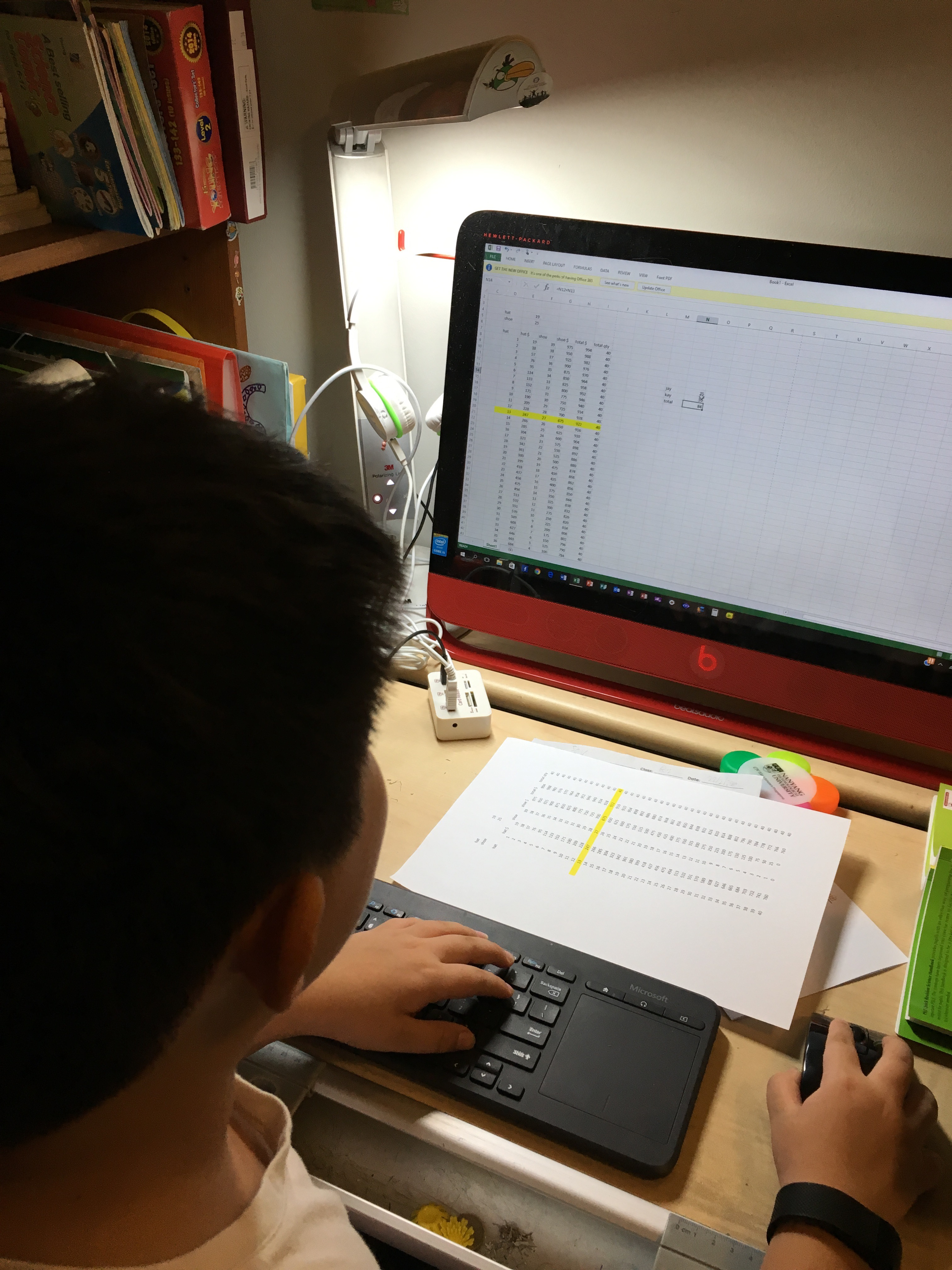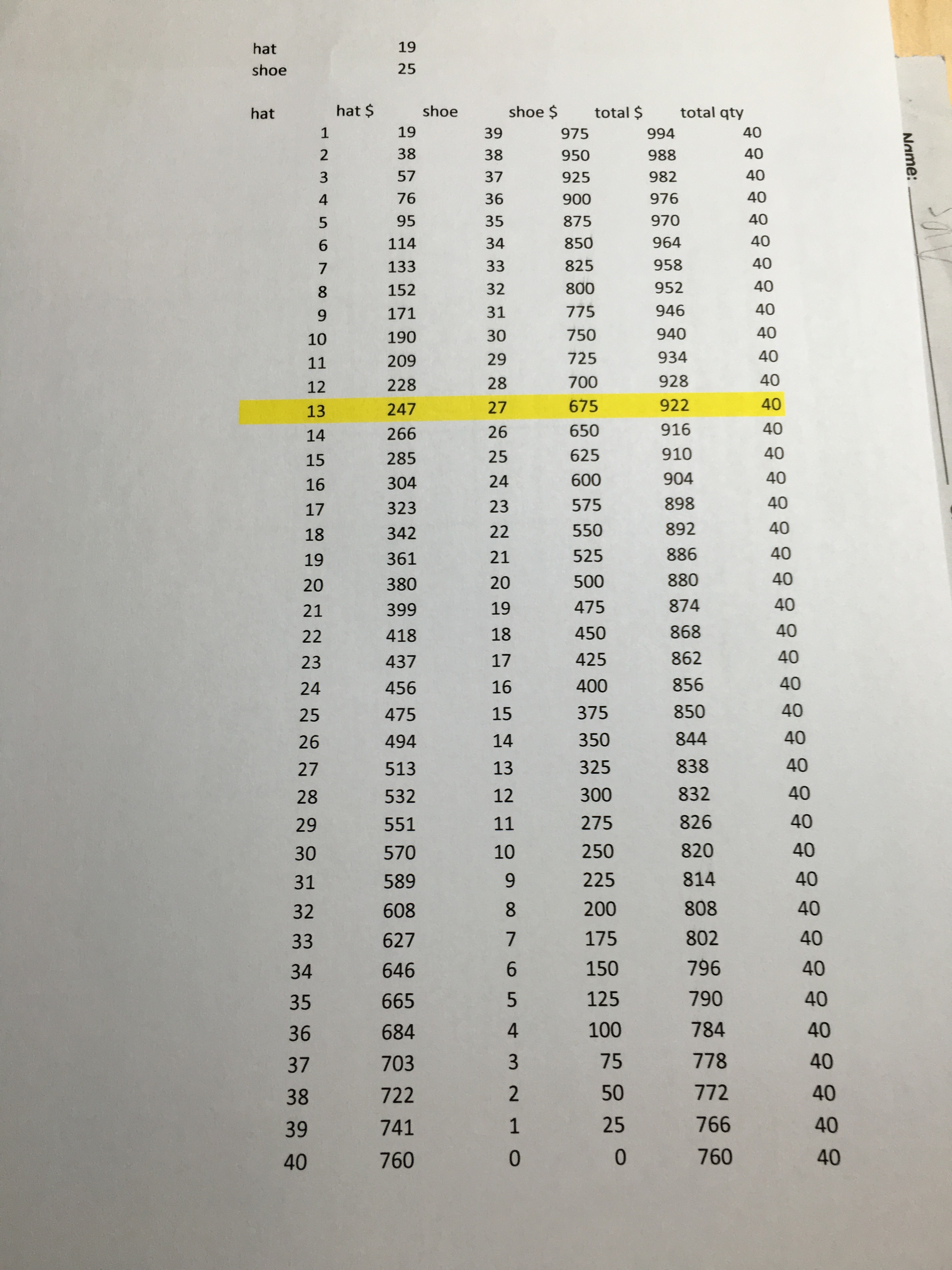Date:  Feb 25, 2016

When your kid is in P4, you wanted him to think out of the cap.  You wanted him to know how to solve a math question effectively and of course, the ways of getting your answers.

This is one of the questions that I hated to do, because it is very tedious.

I simply walked by and saw Jay doing this question.  And he started at the right place, but continue and the wrong direction.

So, you have \$922, and you can buy only 40 in total quantity of either hats or shoes.  Each hat is \$19, and the shoe cost \$25.  So, you need to find the perfect match that sums up to \$922.Yes, he started from the half, work out the 20 hats and 20 shoe cost.  Unfortunately, he works downward, instead of upward.  Because when you find the total of 20 hats and 20 shoes is \$880, and you need to buy more expensive stuffs to make the sums close to \$922.  So, he should have done is working upwards, i.e. 21 shoes and 19 hats and so on.  But he didn’t.

Hahaha, well, some times when your mind got stuck, you will need to lend it a hand.  This is where I come in.

So, I quickly launch the Excel spreadsheet and try to work out the answers using computer.

Jay was amazed, and eager to learn what I was doing.  This is the first time he learned how to enter numbers into the spreadsheets and how to use formulas to do the sums.  And by dragging the formula across all the rows, all the answers are reflected immediately.

And this gives me a chance to change the cost of shoes and hats, and a refreshed total and sums are shown.And after seeing the print out of this nicely done spreadsheet, he finally get what I mean.  And I am very sure that from now on, when he sees this kind of questions, he knows that he does not need to work out so many numbers to find the answer.

By looking at the “cheat sheet”, he learned

1. start at half way, 20 shoes and 20 hats.  This gives \$880.
2. look at the total for 20 shoes and 20 hats, see if the total is greater or lower than \$922.
3. If it is lower, then, you have to buy more expensive shoes, i.e. \$25.
4. So, you work out 21 shoes and 19 hats.  That gives \$886.
5. So, you figure out the difference each time is 6 if you increase one shoe and decrease one hat.  i.e. 22 shoes and 18 hats will give \$886 + 6 = \$892.
6. So, if \$922 is where we want to go, \$922 – \$880 = \$42.  so, 7 x \$6 = \$42.  And you get your answers. 27 shoes and 13 hats.I think P4 is a right age to introduce them with excel spreadsheet.  Life is about problem solving.  They will learn a set of skills set according to MOE syllabus from school.  And at home, you can teach them other skill sets so to make it a complete.

I love teaching my kids.  🙂